×

Search anything:

# Text Summarization using RNN

#### Machine Learning (ML) Natural Language Processing (NLP)FREE BOOK -> Problems for the day before your Coding Interview (on Amazon)

Reading time: 40 minutes | Coding time: 15 minutes

Encoder Decoder RNN (Recurrent neural network) model is used in order to overcome all the limits faced by the NLP for text summarization such as getting a short and accurate summary. It is a much more intelligent and smart approach.

We have explored this in depth in this article at OpenGenus IQ.

# Introduction

We can convert a document summmarization problem into a supervised and semi-supervised machine learning problem. Mostly we use sequence-2-sequence architecture of RNN . A sequence2sequence model basically comprises of an Encoder and a Decoder connected sequentially so as to generate an output (Summary) for a given input (Text).

## RNN for text summarization

In RNN, the new output is dependent on previous output. Due to this property of RNN we try to summarize our text as more human like as possible.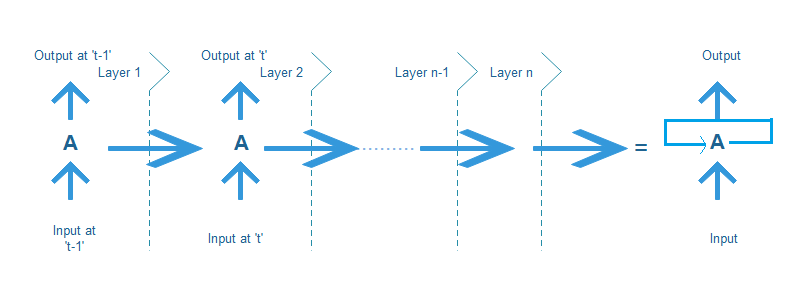Training: Recurrent neural network use back propagation algorithm, but it is applied for every time stamp. It is commonly known as backpropagation through time(BTT).
We use a Sequence2Sequence model comprising of Encoders and Decoders to summarize our long input statements into a summarized line. These Encoders and decoders further comrpises of LSTM's(discussed later) as an extended functionality of a simple RNN.

## Computations for simple RNN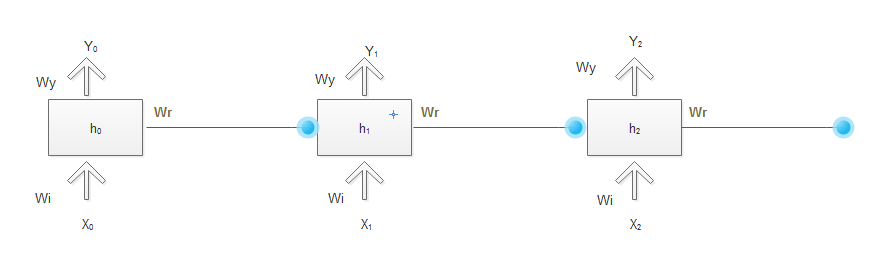h(t)=gh(Wi.x(t)+Wrh(t-1)+bh)
y(t)=gy(Wy.h(t)+by)

Where X denotes the input ,Y denotes output followed by subscript denoting the time. h(t) is the processing function and y(t) is the output function. g() represents the function determining the h(t) and y(t).

## LSTM's(Long-Short term memory network)

It is a special kind of RNN. Which is capable of learning long term dependencies thus treating the problem of short term dependencies of a simple RNN. It is not possible for a RNN to remember the to understand the context behind the input while we try to achieve this using a LSTM.

The tanh layer is used as a squashing function i.e. it converts the values in [-1,1].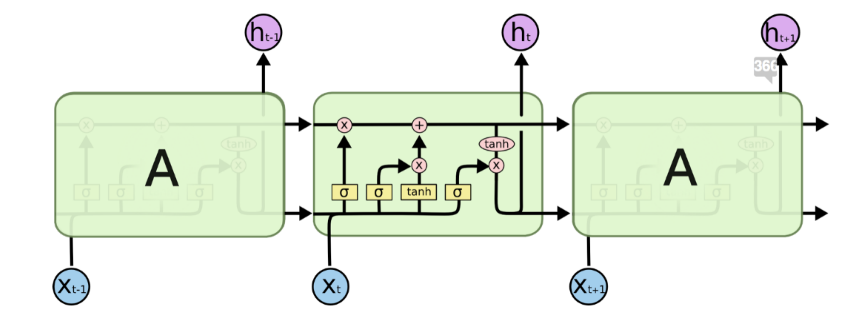Here identify the information that is not required and will be thrown away from cell state. This decision is made by sigmoid layer (as shown in figure) which is also known as forget layer. Using the next sigmoid layer we try to determine the new information that we are going to store. In this process, a sigmoid layer known as input gate layer decides which values will be updated.
In the next step, we update the old cell state Ct-1 into Ct thus determining the new candidate values.

Ct=ft*Ct-1+it*Ct

In the last step we run a sigmoid layer which describes the output.

Ot=sigma(Wo[ht-1,xt]+bo)

where xt=New input,
bf=bias,
wf=weight,
ht-1=Output from previous time stamp
and ft=sigm(wf[ht-1,xt]+bf)

## Encoder-Decoder Architecture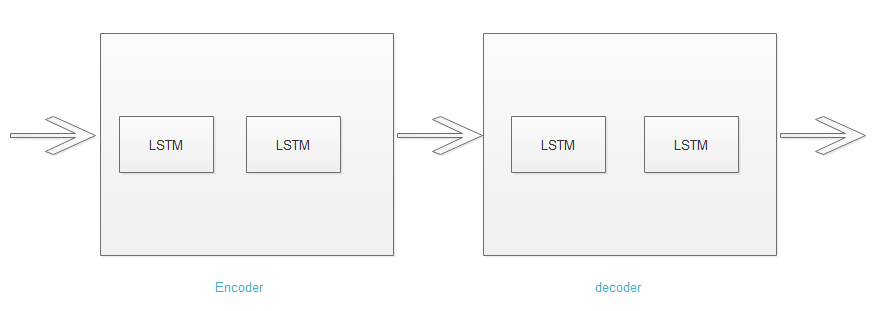Encoder and Decoders are the two components of a sequence-2-sequence rnn architecture. These are majorly used when inputs and outputs are of varying lengths. In this architecture, various LSTMs are placed sequentially in order to encode and then decode the input.

Encoder - It encodes the entire input into a form suitable for processing.
Decoder - It decodes the processed input into an understable and required output.

One word at a time is provided as input to the encoder with its time stamp. This word is then processed in the LSTM by retieving the information present in the sequenced input. It is followed by a decoder that decodes the input sequence into a more readable and desirable output format. It is also dependent upon the time stamp. Finally, we produce a summary using the model which predicts the next relecant word by considering the previous sequence of words.

This model is an extension to feedforward network with atleast one feedback connection. In standard LSTM sequence of fixed length is passed as an input to be encoded into a fixed dimension vector (v), which is then decoded into the output sequence of words.

1. Generates more human like summary.
2. Accuracy increases with the data size.

1. Complex model
2. Repetition of word and phases is not checked.
3. Abstractive summarizers are comparitively slower.

## Model summarization

For implementing our neural network we would use keras library of python along with other libraries required for data preprocessing and designing. After loading the data we use the contraction mapping in order to deal with the contracted words of the language. It helps in better understanding(intended meaning) and improved accuracy of the model.

Moving on to the data preprocessing step, data cleaning is implemented along with removal of stopwords in order to make data ready for our model. This phase is implemented separately for both summary and complete text of the training model.
In the next phase model building is implemented. 3 Layers of LSTM are added(can be increased or decreased accordingly) along with encoder and decoder.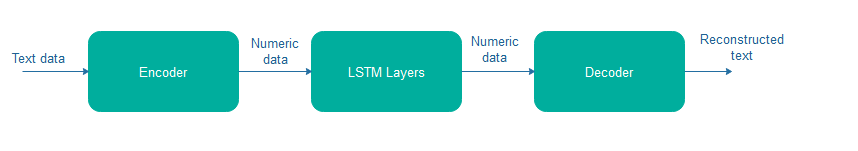It is followed by attention layer(discussed in pseudocode) and a dense layer before the compilation phase. Finally we complete the summarization using the data generated and adding it sequentially using the decode_seq method and seq2seq method.
It if followed by seq2text method to add the text into the sequence. In the end, we have our required summary.

## Implementation

Import the required libraries. Tensorflow and keras are the main libraries that we use in order to implement RNN along with other essential libraries.

``````import numpy as np
import pandas as pd
import re
from bs4 import BeautifulSoup
from keras.preprocessing.text import Tokenizer
from nltk.corpus import stopwords
from tensorflow.keras.layers import Input, LSTM, Embedding, Dense, Concatenate, TimeDistributed, Bidirectional
from tensorflow.keras.models import Model
from tensorflow.keras.callbacks import EarlyStopping
import warnings
``````

Read the data and preprocess the data.
We use contraction_mapping dictionary in order to map the contracted words with their intended meanings.

``````data=pd.read_csv("reviews.csv")
contraction_map = {"ain't": "is not", "aren't": "are not","can't": "cannot", "'cause": "because", "could've": "could have", "couldn't": "could not",

"didn't": "did not", "doesn't": "does not", "don't": "do not", "hadn't": "had not", "hasn't": "has not", "haven't": "have not",

"he'd": "he would","he'll": "he will", "he's": "he is", "how'd": "how did", "how'd'y": "how do you", "how'll": "how will", "how's": "how is",

"I'd": "I would", "I'd've": "I would have", "I'll": "I will", "I'll've": "I will have","I'm": "I am", "I've": "I have", "i'd": "i would",

"i'd've": "i would have", "i'll": "i will",  "i'll've": "i will have","i'm": "i am", "i've": "i have", "isn't": "is not", "it'd": "it would",

"it'd've": "it would have", "it'll": "it will", "it'll've": "it will have","it's": "it is", "let's": "let us", "ma'am": "madam",

"mayn't": "may not", "might've": "might have","mightn't": "might not","mightn't've": "might not have", "must've": "must have",

"mustn't": "must not", "mustn't've": "must not have", "needn't": "need not", "needn't've": "need not have","o'clock": "of the clock",

"oughtn't": "ought not", "oughtn't've": "ought not have", "shan't": "shall not", "sha'n't": "shall not", "shan't've": "shall not have",

"she'd": "she would", "she'd've": "she would have", "she'll": "she will", "she'll've": "she will have", "she's": "she is",

"should've": "should have", "shouldn't": "should not", "shouldn't've": "should not have", "so've": "so have","so's": "so as",

"this's": "this is","that'd": "that would", "that'd've": "that would have", "that's": "that is", "there'd": "there would",

"there'd've": "there would have", "there's": "there is", "here's": "here is","they'd": "they would", "they'd've": "they would have",

"they'll": "they will", "they'll've": "they will have", "they're": "they are", "they've": "they have", "to've": "to have",

"wasn't": "was not", "we'd": "we would", "we'd've": "we would have", "we'll": "we will", "we'll've": "we will have", "we're": "we are",

"we've": "we have", "weren't": "were not", "what'll": "what will", "what'll've": "what will have", "what're": "what are",

"what's": "what is", "what've": "what have", "when's": "when is", "when've": "when have", "where'd": "where did", "where's": "where is",

"where've": "where have", "who'll": "who will", "who'll've": "who will have", "who's": "who is", "who've": "who have",

"why's": "why is", "why've": "why have", "will've": "will have", "won't": "will not", "won't've": "will not have",

"would've": "would have", "wouldn't": "would not", "wouldn't've": "would not have", "y'all": "you all",

"y'all'd": "you all would","y'all'd've": "you all would have","y'all're": "you all are","y'all've": "you all have",

"you'd": "you would", "you'd've": "you would have", "you'll": "you will", "you'll've": "you will have",

"you're": "you are", "you've": "you have"}
``````

Remove the stop-words and store the cleaned data in a separate file.

``````stop_words = set(stopwords.words('english'))
def text_cleaner(text):
newString = text.lower()
newString = BeautifulSoup(newString, "lxml").text
newString = re.sub(r'\([^)]*\)', '', newString)
newString = re.sub('"','', newString)
newString = ' '.join([contraction_mapping[t] if t in contraction_mapping else t for t in newString.split(" ")])
newString = re.sub(r"'s\b","",newString)
newString = re.sub("[^a-zA-Z]", " ", newString)
tokens = [w for w in newString.split() if not w in stop_words]
long_words=[]
for i in tokens:
if len(i)>=3:                  #removing short word
long_words.append(i)
return (" ".join(long_words)).strip()

cleaned_text = []
for t in data['Text']:
cleaned_text.append(text_cleaner(t))
``````

Similarly, clean the summary using the contrcation_mapping and removing irrelevant letters and words. Finally,tokenize the sentences for further processing.

``````def summary_cleaner(text):
newString = re.sub('"','', text)
newString = ' '.join([contraction_mapping[t] if t in contraction_mapping else t for t in newString.split(" ")])
newString = re.sub(r"'s\b","",newString)
newString = re.sub("[^a-zA-Z]", " ", newString)
newString = newString.lower()
tokens=newString.split()
newString=''
for i in tokens:
if len(i)>1:
newString=newString+i+' '
return newString

#Call the above function
cleaned_summary = []
for t in data['Summary']:
cleaned_summary.append(summary_cleaner(t))

data['cleaned_text']=cleaned_text
data['cleaned_summary']=cleaned_summary
data['cleaned_summary'].replace('', np.nan, inplace=True)
data.dropna(axis=0,inplace=True)
``````

Set the lengths for summary and text.

``````max_len_text=80
max_len_summary=10
``````

Build the model. Key components of the model are as follows:

• Encoder- Encoder_inputs is used in order to encode the words into numeric data for processing by LSTM layers.
• LSTM Layers- We use 3 LSTM layers in order to process the data effectively. You can also experiment by adding or removing the layers in order to find more better accuracies. return_sequences in LSTM layer is set true until we want to add more layers consecutively.
• Decoder- Decoder again converts the numeric data into the understandable word formats.
``````from sklearn.model_selection import train_test_split
x_tr,x_val,y_tr,y_val=train_test_split(data['cleaned_text'],data['cleaned_summary'],test_size=0.1,random_state=0,shuffle=True)
from keras import backend as K
K.clear_session()
latent_dim = 500

# Encoder
encoder_inputs = Input(shape=(max_len_text,))
enc_emb = Embedding(x_voc_size, latent_dim,trainable=True)(encoder_inputs)

#Preparing LSTM layer 1
encoder_lstm1 = LSTM(latent_dim,return_sequences=True,return_state=True)
encoder_output1, state_h1, state_c1 = encoder_lstm1(enc_emb)

#Preparing LSTM layer 2
encoder_lstm2 = LSTM(latent_dim,return_sequences=True,return_state=True)
encoder_output2, state_h2, state_c2 = encoder_lstm2(encoder_output1)

#Preparing LSTM layer 3
encoder_lstm3=LSTM(latent_dim, return_state=True, return_sequences=True)
encoder_outputs, state_h, state_c= encoder_lstm3(encoder_output2)

# Decoder layer
decoder_inputs = Input(shape=(None,))
dec_emb_layer = Embedding(y_voc_size, latent_dim,trainable=True)
dec_emb = dec_emb_layer(decoder_inputs)

decoder_lstm = LSTM(latent_dim, return_sequences=True, return_state=True)
decoder_outputs,decoder_fwd_state, decoder_back_state = decoder_lstm(dec_emb,initial_state=[state_h, state_c])
``````

Adding the Attention layer: Attention layer is used to selectively choose the relevant information while discarding the non-useful information by cognitively mapping the generated sentences with the inputs of encoder layer.
Dense Layer: It mathematically represents the matrix vector multiplication in neurons and is used to change the dimensions of the vectors for processing between various layers.

``````#Preparing the Attention Layer
Attention layer attn_layer = AttentionLayer(name='attention_layer')
attn_out, attn_states = attn_layer([encoder_outputs, decoder_outputs])
decoder_concat_input = Concatenate(axis=-1, name='concat_layer')([decoder_outputs, attn_out])

decoder_dense = TimeDistributed(Dense(y_voc_size, activation='softmax'))
decoder_outputs = decoder_dense(decoder_concat_input)

# Prepare the model
model = Model([encoder_inputs, decoder_inputs], decoder_outputs)

# Compiling the RNN model
model.compile(optimizer='rmsprop', loss='sparse_categorical_crossentropy')
``````

Decode the decoder sequence for text generation and convert the integer sequence into word sequence for summary.

``````def decode_sequence(input_sequence):
# Encode the input as state vectors.
e_out, e_h, e_c = encoder_model.predict(input_sequence)

# Generate empty target sequence of length 1.
target_seq = np.zeros((1,1))

# Chose the 'start' word as the first word of the target sequence
target_seq[0, 0] = target_word_index['start']

stop_condition = False
decoded_sentence = ''
while not stop_condition:
output_tokens, h, c = decoder_model.predict([target_seq] + [e_out, e_h, e_c])

# Sample a token
sampled_token_index = np.argmax(output_tokens[0, -1, :])
sampled_token = reverse_target_word_index[sampled_token_index]

if(sampled_token!='end'):
decoded_sentence += ' '+sampled_token

# Exit condition: either hit max length or find stop word.
if (sampled_token == 'end' or len(decoded_sentence.split()) >= (max_len_summary-1)):
stop_condition = True

# Update the target sequence (of length 1).
target_seq = np.zeros((1,1))
target_seq[0, 0] = sampled_token_index

# Update internal states
e_h, e_c = h, c

return decoded_sentence
## Making the seq2seq summary
def seq2seqsummary(input_sequence):
newString=''
for i in input_sequence:
if((i!=0 and i!=target_word_index['start']) and i!=target_word_index['end']):
newString=newString+reverse_target_word_index[i]+' '
return newString

def seq2text(input_sequence):
newString=''
for i in input_sequence:
if(i!=0):
newString=newString+reverse_source_word_index[i]+' '
return newString
``````

Output can be predicted using the simple for function.

``````for i in range(len(x_val)):
print("Predicted summary:",decode_sequence(x_val[i].reshape(1,max_len_text)))
print("\n")
``````

## Output

Input text

1. Microsoft chairman bill gates late wednesday unveiled his vision of the digital lifestyle , outlining the latest version of his windows operating system to be launched later this year .
2. The final results of iraq 's december general elections are due within the next four days , a member of the iraqi electoral commission said on thursday ."

Summary

1. Bill gates unveils new technology vision.
2. Iraqi election results due in next four days.

Titles by humans

1. Gates unveils microsoft 's vision of digital lifestyle
2. Iraqi election final results out within four days